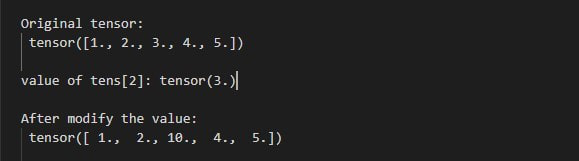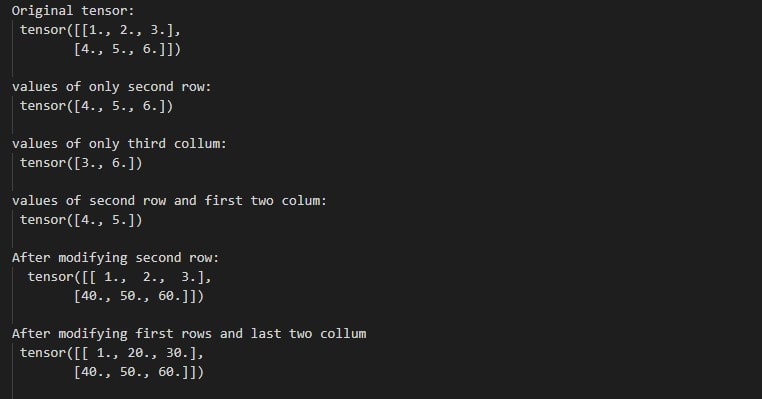GeeksforGeeks App
Open AppBrowser
Continue

# How to access and modify the values of a Tensor in PyTorch?

In this article, we are going to see how to access and modify the value of a tensor in PyTorch using Python.

We can access the value of a tensor by using indexing and slicing. Indexing is used to access a single value in the tensor. slicing is used to access the sequence of values in a tensor. we can modify a tensor by using the assignment operator. Assigning a new value in the tensor will modify the tensor with the new value.

Import the torch libraries and then create a PyTorch tensor. Access values of the tensor. Modify a value with a new value by using the assignment operator.

Example 1: Access and modify value using indexing. in the below example, we are accessing and modifying the value of a tensor.

## Python

 `# Import torch libraries``import` `torch` `# create PyTorch tensor``tens ``=` `torch.Tensor([``1``, ``2``, ``3``, ``4``, ``5``])` `# print tensor``print``(``"Original tensor:"``, tens)` `# access a value by their index``temp ``=` `tens[``2``]``print``(``"value of tens:"``, temp)`  `# modify a value.``tens[``2``] ``=` `10` `# print tensor after modify the value``print``(``"After modify the value:"``, tens)`

Output:Example 2:  Access and modify the sequence of values in tensor using slicing.

## Python

 `# Import torch libraries``import` `torch` `# create PyTorch Tensor``tens ``=` `torch.Tensor([[``1``, ``2``, ``3``], [``4``, ``5``, ``6``]])` `# Print the tensor``print``(``"Original tensor: "``, tens)`  `# Access all values of only second row``# using slicing``a ``=` `tens[``1``]``print``(``"values of only second row: "``, a)` `# Access all values of only third column``b ``=` `tens[:, ``2``]``print``(``"values of only third column: "``, b)` `# Access values of second row and first``# two column``c ``=` `tens[``1``, ``0``:``2``]``print``(``"values of second row and first two column: "``, c)` `# Modifying all the values of second row``tens[``1``] ``=` `torch.Tensor([``40``, ``50``, ``60``])``print``(``"After modifying second row:  "``, tens)` `# Modify values of first rows and last``# two column``tens[``0``, ``1``:``3``] ``=` `torch.Tensor([``20``, ``30``])``print``(``"After modifying first rows and last two column "``, tens)`

Output:My Personal Notes arrow_drop_up